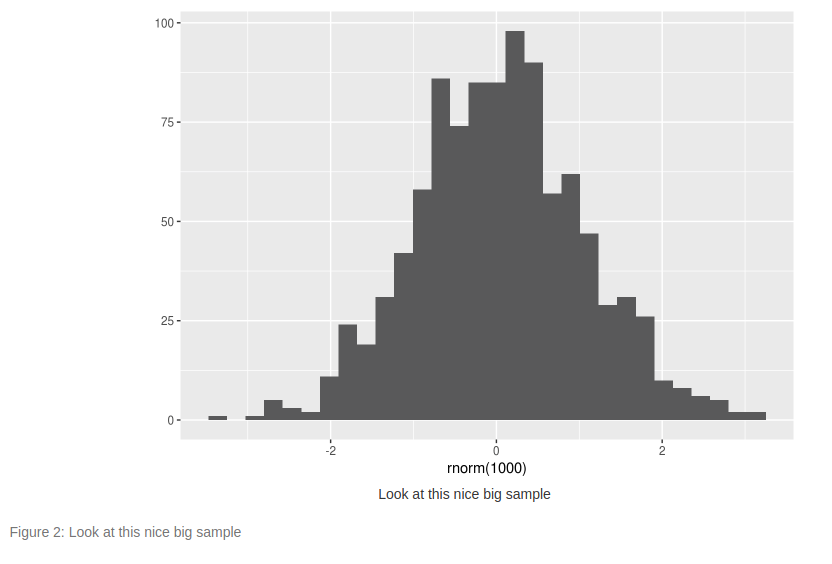Instantly share code, notes, and snippets.benmarwick/captions_and_crossrefs.rmd Last active Sep 30, 2019

Auto-numbering and cross-referencing of figures and tables in rmarkdown
 --- title: "Auto-numbering and cross-referencing of figures and tables in rmarkdown" output: html_document --- NOTE: I recommend using the bookdown package and `output: html_document2` to make captions and cross-references more easily than the method described below. TODO: check this out: https://github.com/adletaw/captioner Here's how to use: 1. Copy and paste the first two code chunks in this document to the top of your document 2. Make sure you have knitr and kfigr installed, use `devtools::install_github("github mkoohafkan/kfigr")` to install kfigr 3. For chunks in your doc that produce a figure or table for which you want a caption, be sure to include a label in the chuck options line. For example, the first chunk is this doc has the label `number_figures` 4. Also in the chunk options line, you need to specify the anchor type and the caption, for example `anchor="figure", fig.cap="Here is a nice figure"`, see the examples below. That will auto-generate the caption and give you auto-numering. See the example below for using with tables. 5. When you want to refer to the figure or table in your text, use a bit of in-line code like so `r figr('my-label', TRUE, type="figure")` which will generate a cross-reference like 'figure 3' or whatever the correct number is for the figure produced by the chunk that has the label 'my-label' 6. Other similar methods: - http://rmflight.github.io/posts/2012/10/papersinRmd.html & https://gist.github.com/rmflight/3858973 - http://galahad.well.ox.ac.uk/repro/ - http://gforge.se/2014/01/fast-track-publishing-using-knitr-part-iii/ ```{r number_figures, echo=FALSE} # This is a knitr hook to enable figure and table captions # number figures # from http://stackoverflow.com/a/18672268/1036500 library("knitr") tn = local({ i = 0 function(x) { i <<- i + 1 paste("", 'Figure ', i, ': ', x, "

", sep = '') } }) knit_hooks\$set(fig.cap = function(before, options, envir) { if(!before) tn(options\$fig.cap) }) default_output_hook = knit_hooks\$get("output") knit_hooks\$set(output = function(x, options) { if (is.null(options\$fig.cap) == F) x else default_output_hook(x,options) }) # number tables # from http://stackoverflow.com/a/18672268/1036500 to = local({ i = 0 function(x) { i <<- i + 1 paste("

", 'Table ', i, ': ', x, "

", sep = '') } }) knit_hooks\$set(tab.cap = function(before, options, envir) { if(!before) to(options\$tab.cap) }) default_output_hook = knit_hooks\$get("output") knit_hooks\$set(output = function(x, options) { if (is.null(options\$tab.cap) == F) x else default_output_hook(x,options) }) ``` ```{r setup, echo=FALSE, message=FALSE} require(knitr) require(kfigr) # devtools::install_github("github mkoohafkan/kfigr") opts_chunk\$set(message=FALSE, warning=FALSE) ``` # My document starts here We can embed plots, for example: ```{r first, anchor="figure", fig.cap="Rather small sample", fig.align='center'} require(ggplot2) qplot(rnorm(10), geom="histogram") ``` Note that for `r figr('first', TRUE, type="figure")` we can refer to the figure by the chunck label and that will generate an auto-numbered reference in the text that also links to the figure. ```{r second, anchor="figure", fig.cap="Look at this nice big sample", fig.align='center'} require(ggplot2) qplot(rnorm(1000), geom="histogram") ``` Note that for `r figr('second', TRUE, type="figure")` we can do the same thing, numbering is taken care of for us. ```{r mytable, anchor="table", tab.cap="my table about cars", tab.align='center'} kable(mtcars) ``` And we can have a table, see `r figr('mytable', TRUE, type="table")` we can refer to the table with a numbered reference in the text that also links to the table. We can refer to the figures and tables multiple times in the text with no problems: `r figr('first', TRUE, type="figure")`, `r figr('second', TRUE, type="figure")`, `r figr('mytable', TRUE, type="table")`

amm03 commented Aug 15, 2018 • edited

 This works great for me with word so far. Modified it so i get Figure S#. instead of figure #. However, in the in line text reference i get Figure S # instead of the desired Figure S#. How can i remove the space between S and # in the cross reference in the text? Thank you in advance for your help and contribution with this.

BroVic commented Aug 27, 2018

 This is great. Thanks for the contribution!

nmolanog commented Sep 23, 2018

 For figures the title in fig.cap appears twice in the document:nmolanog commented Sep 23, 2018 • edited

 I found an issue: if you add the following chunck to your document summary(mtcars) you get the following error Error: C stack usage 7969380 is too close to the limit Execution halted I found this issue working on my own documents using your functions and took me a while to find this.
to join this conversation on GitHub. Already have an account? Sign in to comment
You can’t perform that action at this time.